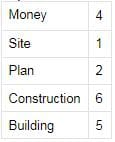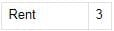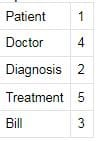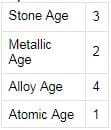Test: Logical Sequence- 4

# Test: Logical Sequence- 4

Test Description

## 30 Questions MCQ Test CSAT Preparation for UPSC CSE | Test: Logical Sequence- 4

Test: Logical Sequence- 4 for CDS / AFCAT 2022 is part of CSAT Preparation for UPSC CSE preparation. The Test: Logical Sequence- 4 questions and answers have been prepared according to the CDS / AFCAT exam syllabus.The Test: Logical Sequence- 4 MCQs are made for CDS / AFCAT 2022 Exam. Find important definitions, questions, notes, meanings, examples, exercises, MCQs and online tests for Test: Logical Sequence- 4 below.
Solutions of Test: Logical Sequence- 4 questions in English are available as part of our CSAT Preparation for UPSC CSE for CDS / AFCAT & Test: Logical Sequence- 4 solutions in Hindi for CSAT Preparation for UPSC CSE course. Download more important topics, notes, lectures and mock test series for CDS / AFCAT Exam by signing up for free. Attempt Test: Logical Sequence- 4 | 30 questions in 30 minutes | Mock test for CDS / AFCAT preparation | Free important questions MCQ to study CSAT Preparation for UPSC CSE for CDS / AFCAT Exam | Download free PDF with solutions
 1 Crore+ students have signed up on EduRev. Have you?
Test: Logical Sequence- 4 - Question 1

### Directions to Solve In each of the following questions, arrange the given words in a meaningful sequence and thus find the correct answer from alternatives. Question -   Arrange the words given below in a meaningful sequence.    1. Rain    2. Monsoon    3. Rescue    4. Flood    5. Shelter    6. Relief

Test: Logical Sequence- 4 - Question 2

### Directions to Solve In each of the following questions, arrange the given words in a meaningful sequence and thus find the correct answer from alternatives. Question -   Arrange the words given below in a meaningful sequence.    1. Foetus    2. Child    3. Baby    4. Adult    5. Youth

Test: Logical Sequence- 4 - Question 3

### Directions to Solve In each of the following questions, arrange the given words in a meaningful sequence and thus find the correct answer from alternatives. Question -   Arrange the words given below in a meaningful sequence.    1. Sea    2. Rivulet    3. Ocean    4. River    5. Glacier

Test: Logical Sequence- 4 - Question 4

Directions to Solve

In each of the following questions, arrange the given words in a meaningful sequence and thus find the correct answer from alternatives.

Question -

Arrange the words given below in a meaningful sequence.

1. Milky way    2. Sun    3. Moon    4. Earth    5. Stars

Detailed Solution for Test: Logical Sequence- 4 - Question 4

The words given are different planetary bodies.
They must be arranged in the increasing or decreasing order of their sizes.
So for given words, the order will be:
Moon, Earth, Sun, Stars, MilkyWay

Test: Logical Sequence- 4 - Question 5

Directions to Solve

In each of the following questions, arrange the given words in a meaningful sequence and thus find the correct answer from alternatives.

Question -

Arrange the words given below in a meaningful sequence.

1. Doctor    2. Fever    3. Prescribe    4. Diagnose    5. Medicine

Test: Logical Sequence- 4 - Question 6

Directions to Solve

In each of the following questions, arrange the given words in a meaningful sequence and thus find the correct answer from alternatives.

Question -

Arrange the words given below in a meaningful sequence.

1. Reading    2. Composing    3. Writing    4. Printing

Detailed Solution for Test: Logical Sequence- 4 - Question 6

The words given are the various stages in making of a book.
So first step is the Writing.
Next will be Composing it suitably.
Then comes Printing and finally it is read by the reader.
so order is: 3,2,4,1

Test: Logical Sequence- 4 - Question 7

Directions to Solve

In each of the following questions, arrange the given words in a meaningful sequence and thus find the correct answer from alternatives.

Question -

Arrange the words given below in a meaningful sequence.

1. Hecto    2. Centi    3. Deca    4. Kilo    5. Deci

Detailed Solution for Test: Logical Sequence- 4 - Question 7

The correct order is :
Centi    2
Deci    5
Deca    3
Hecto    1
Kilo    4

Test: Logical Sequence- 4 - Question 8

Directions to Solve

In each of the following questions, arrange the given words in a meaningful sequence and thus find the correct answer from alternatives.

Question -

Arrange the words given below in a meaningful sequence.

1. Honey    2. Flower    3. Bee    4. Wax

Test: Logical Sequence- 4 - Question 9

Directions to Solve

In each of the following questions, arrange the given words in a meaningful sequence and thus find the correct answer from alternatives.

Question -

Arrange the words given below in a meaningful sequence.

1. Probation    2. Interview    3. Selection

Test: Logical Sequence- 4 - Question 10

Directions to Solve

In each of the following questions, arrange the given words in a meaningful sequence and thus find the correct answer from alternatives.

Question -

Arrange the words given below in a meaningful sequence.

1. Country    2. Furniture    3. Forest    4. Wood    5. Trees

Test: Logical Sequence- 4 - Question 11

Directions to Solve

In each of the following questions, arrange the given words in a meaningful sequence and thus find the correct answer from alternatives.

Question -

Arrange the words given below in a meaningful sequence.

1. Site    2. Plan    3. Rent    4. Money    5. Building    6. Construction

Detailed Solution for Test: Logical Sequence- 4 - Question 11

The correct order is :Test: Logical Sequence- 4 - Question 12

Directions to Solve

In each of the following questions, arrange the given words in a meaningful sequence and thus find the correct answer from alternatives.

Question -

Arrange the words given below in a meaningful sequence.

1. Key    2. Door    3. Lock    4. Room    5. Switch on

Test: Logical Sequence- 4 - Question 13

Directions to Solve

In each of the following questions, arrange the given words in a meaningful sequence and thus find the correct answer from alternatives.

Question -

Arrange the words given below in a meaningful sequence.

1. District    2. Village    3. State    4. Town    5. City

Test: Logical Sequence- 4 - Question 14

Directions to Solve

In each of the following questions, arrange the given words in a meaningful sequence and thus find the correct answer from alternatives.

Question -

Arrange the words given below in a meaningful sequence.

1. Rainbow    2. Rain    3. Sun    4. Happy    5. Child

Test: Logical Sequence- 4 - Question 15

Directions to Solve

In each of the following questions, arrange the given words in a meaningful sequence and thus find the correct answer from alternatives.

Question -

Arrange the words given below in a meaningful sequence.

1. Heel    2. Shoulder    3. Skull    4. Neck    5. Knee

6. Chest    7. Thigh    8. Stomach    9. Face    10. Hand

Test: Logical Sequence- 4 - Question 16

Directions to Solve

In each of the following questions, arrange the given words in a meaningful sequence and thus find the correct answer from alternatives.

Question -

Arrange the words given below in a meaningful sequence.

1. Cutting    2. Dish    3. Vegetable    4. Market    5. Cooking

Test: Logical Sequence- 4 - Question 17

Directions to Solve

In each of the following questions, arrange the given words in a meaningful sequence and thus find the correct answer from alternatives.

Question -

Arrange the words given below in a meaningful sequence.

1. Elephant    2. Cat    3. Mosquito    4. Tiger    5. Whale

Test: Logical Sequence- 4 - Question 18

Directions to Solve

In each of the following questions, arrange the given words in a meaningful sequence and thus find the correct answer from alternatives.

Question -

Arrange the words given below in a meaningful sequence.

1. Cut    2. Put on    3. Mark    4. Measure    5. Tailor

Test: Logical Sequence- 4 - Question 19

Directions to Solve

In each of the following questions, arrange the given words in a meaningful sequence and thus find the correct answer from alternatives.

Question -

Arrange the words given below in a meaningful sequence.

1. Yarn    2. Plant    3. Saree    4. Cotton    5. Cloth

Test: Logical Sequence- 4 - Question 20

Directions to Solve

In each of the following questions, arrange the given words in a meaningful sequence and thus find the correct answer from alternatives.

Question -

Arrange the words given below in a meaningful sequence.

1. Police    2. Punishment    3. Crime    4. Justice    5. Judgement

Test: Logical Sequence- 4 - Question 21

Directions to Solve

In each of the following questions, arrange the given words in a meaningful sequence and thus find the correct answer from alternatives.

Question -

Arrange the words given below in a meaningful sequence.

1. Patient    2. Diagnosis    3. Bill    4. Doctor    5. Treatment

Detailed Solution for Test: Logical Sequence- 4 - Question 21

The correct order is :Test: Logical Sequence- 4 - Question 22

Directions to Solve

In each of the following questions, arrange the given words in a meaningful sequence and thus find the correct answer from alternatives.

Question -

Arrange the words given below in a meaningful sequence.

1. Andhra Pradesh    2. Universe    3. Tirupati    4. World    5. India

Test: Logical Sequence- 4 - Question 23

Directions to Solve

In each of the following questions, arrange the given words in a meaningful sequence and thus find the correct answer from alternatives.

Question -

Arrange the words given below in a meaningful sequence.

1. Study    2. Job    3. Examination    4. Earn    5. Apply

Test: Logical Sequence- 4 - Question 24

Directions to Solve

In each of the following questions, arrange the given words in a meaningful sequence and thus find the correct answer from alternatives.

Question -

Arrange the words given below in a meaningful sequence.

1. Atomic Age    2. Metallic Age    3. Stone Age    4. Alloy Age

Detailed Solution for Test: Logical Sequence- 4 - Question 24

The correct order is :Test: Logical Sequence- 4 - Question 25

Directions to Solve

In each of the following questions, arrange the given words in a meaningful sequence and thus find the correct answer from alternatives.

Question -

Arrange the words given below in a meaningful sequence.

1. Book    2. Pulp    3. Timber    4. Jungle    5. Paper

Test: Logical Sequence- 4 - Question 26

Directions to Solve

In each of the following questions, arrange the given words in a meaningful sequence and thus find the correct answer from alternatives.

Question -

Arrange the words given below in a meaningful sequence.

1. Sentence    2. Chapter    3. Letter    4. Book    5. Word    6. Paragraph

Test: Logical Sequence- 4 - Question 27

Directions to Solve

In each of the following questions, arrange the given words in a meaningful sequence and thus find the correct answer from alternatives.

Question -

Arrange the words given below in a meaningful sequence.

1. Leaf    2. Fruit    3. Stem    4. Root    5. Flower

Test: Logical Sequence- 4 - Question 28

Directions to Solve

In each of the following questions, arrange the given words in a meaningful sequence and thus find the correct answer from alternatives.

Question -

Arrange the words given below in a meaningful sequence.

1. College    2. Child    3. Salary    4. School    5. Employment

Test: Logical Sequence- 4 - Question 29

Directions to Solve

In each of the following questions, arrange the given words in a meaningful sequence and thus find the correct answer from alternatives.

Question -

Arrange the words given below in a meaningful sequence.

1. Puberty    2. Adulthood    3. Childhood

4. Infancy    5. Senescence    6. Adolescence

Detailed Solution for Test: Logical Sequence- 4 - Question 29

The words given are various phases experienced by a human in his lifetime.
They must be arranged in the order in which they occur.
First comes the Infancy, then the Childhood, then Puberty , followed by Adolescence, Adulthood and finally comes Senescence.
4,3,6,1,2, 5

Test: Logical Sequence- 4 - Question 30

Directions to Solve

In each of the following questions, arrange the given words in a meaningful sequence and thus find the correct answer from alternatives.

Question -

Arrange the words given below in a meaningful sequence.

1. Mother    2. Child    3. Milk    4. Cry    5. Smile

## CSAT Preparation for UPSC CSE

72 videos|64 docs|92 tests
 Use Code STAYHOME200 and get INR 200 additional OFF Use Coupon Code
Information about Test: Logical Sequence- 4 Page
In this test you can find the Exam questions for Test: Logical Sequence- 4 solved & explained in the simplest way possible. Besides giving Questions and answers for Test: Logical Sequence- 4, EduRev gives you an ample number of Online tests for practice

## CSAT Preparation for UPSC CSE

72 videos|64 docs|92 tests